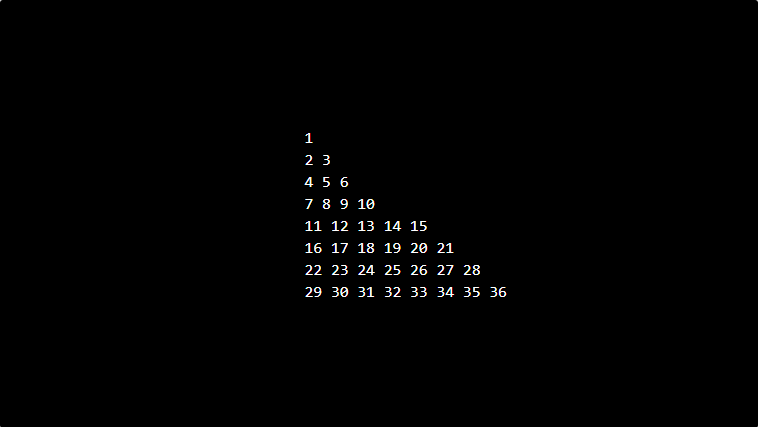# PHP program to print Floyd Triangle number

In this article write a PHP program to print Floyd triangle number. We run for loop 8 times, in which you have 1 outer for loops which contains 1 nested for loop and if condition to print Floyd triangle number as shown in the following program.

## Program to print Floyd triangle number

```<?php
echo "<pre>";
\$num = 1;
for (\$i = 1; \$i <= 8; \$i++) {
for (\$j = 1; \$j <= \$i; \$j++) {
echo \$num . "&nbsp;";
\$num++;
if (\$j == \$i) {
echo "&nbsp;";
echo "<br/>";
}
}
}
echo "</pre>";
?>
```

### Output: